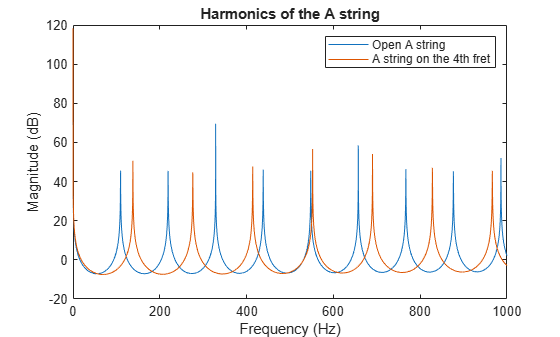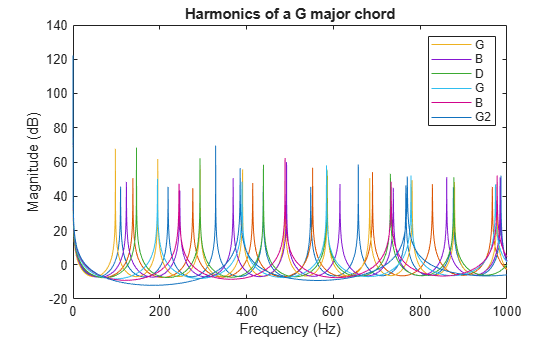Generating Guitar Chords Using the Karplus-Strong Algorithm

This example shows how to generate realistic guitar chords using the Karplus-Strong Algorithm and discrete-time filters.

Setup

Begin by defining variables that we will be using later, e.g. the sampling frequency, the first harmonic frequency of the A string, the offset of each string relative to the A string.

Fs       = 44100;
A        = 110; % The A string of a guitar is normally tuned to 110 Hz
Eoffset  = -5;
Doffset  = 5;
Goffset  = 10;
Boffset  = 14;
E2offset = 19;

Generate the frequency vector that we will use for analysis.

F = linspace(1/Fs, 1000, 2^12);

Generate 4 seconds of zeros to be used to generate the guitar notes.

x = zeros(Fs*4, 1);

Play a Note on an Open String

When a guitar string is plucked or strummed, it produces a sound wave with peaks in the frequency domain that are equally spaced. These are called the harmonics and they give each note a full sound. We can generate sound waves with these harmonics with discrete-time filter objects.

Determine the feedback delay based on the first harmonic frequency.

delay = round(Fs/A);

Generate an IIR filter whose poles approximate the harmonics of the A string. The zeros are added for subtle frequency domain shaping.

b  = firls(42, [0 1/delay 2/delay 1], [0 0 1 1]);
a  = [1 zeros(1, delay) -0.5 -0.5];

Show the magnitude response of the filter.

[H,W] = freqz(b, a, F, Fs);
plot(W, 20*log10(abs(H)));
title('Harmonics of an open A string');
xlabel('Frequency (Hz)');
ylabel('Magnitude (dB)');To generate a 4 second synthetic note first we create a vector of states with random numbers. Then we filter zeros using these initial states. This forces the random states to exit the filter shaped into the harmonics.

zi = rand(max(length(b),length(a))-1,1);
note = filter(b, a, x, zi);

Normalize the sound for the audioplayer.

note = note-mean(note);
note = note/max(abs(note));

% To hear, type: hplayer = audioplayer(note, Fs); play(hplayer)

Play a Note on a Fretted String

Each fret along a guitar's neck allows the player to play a half tone higher, or a note whose first harmonic is ${2}^{1/12}$ higher.

fret  = 4;
delay = round(Fs/(A*2^(fret/12)));

b  = firls(42, [0 1/delay 2/delay 1], [0 0 1 1]);
a  = [1 zeros(1, delay) -0.5 -0.5];

[H,W] = freqz(b, a, F, Fs);
hold on
plot(W, 20*log10(abs(H)));
title('Harmonics of the A string');
legend('Open A string', 'A string on the 4th fret');Populate the states with random numbers.

zi = rand(max(length(b),length(a))-1,1);

Create a 4 second note.

note = filter(b, a, x, zi);

Normalize the sound for the audioplayer.

note = note-mean(note);
note = note/max(note);

% To hear, type: hplayer = audioplayer(note, Fs); play(hplayer)

Play a Chord

A chord is a group of notes played together whose harmonics enforce each other. This happens when there is a small integer ratio between the two notes, e.g. a ratio of 2/3 would mean that the first notes third harmonic would align with the second notes second harmonic.

Define the frets for a G major chord.

fret = [3 2 0 0 0 3];

Get the delays for each note based on the frets and the string offsets.

delay = [round(Fs/(A*2^((fret(1)+Eoffset)/12))), ...
round(Fs/(A*2^(fret(2)/12))), ...
round(Fs/(A*2^((fret(3)+Doffset)/12))), ...
round(Fs/(A*2^((fret(4)+Goffset)/12))), ...
round(Fs/(A*2^((fret(5)+Boffset)/12))), ...
round(Fs/(A*2^((fret(6)+E2offset)/12)))];

b = cell(length(delay),1);
a = cell(length(delay),1);
H = zeros(length(delay),4096);
note = zeros(length(x),length(delay));
for indx = 1:length(delay)

% Build a cell array of numerator and denominator coefficients.
b{indx} = firls(42, [0 1/delay(indx) 2/delay(indx) 1], [0 0 1 1]).';
a{indx} = [1 zeros(1, delay(indx)) -0.5 -0.5].';

% Populate the states with random numbers and filter the input zeros.
zi = rand(max(length(b{indx}),length(a{indx}))-1,1);

note(:, indx) = filter(b{indx}, a{indx}, x, zi);

% Make sure that each note is centered on zero.
note(:, indx) = note(:, indx)-mean(note(:, indx));

[H(indx,:),W] = freqz(b{indx}, a{indx}, F, Fs);
end

Display the magnitude for all the notes in the chord.

hline = plot(W,20*log10(abs(H.')));
title('Harmonics of a G major chord');
xlabel('Frequency (Hz)');
ylabel('Magnitude (dB)');
legend(hline,'G','B','D','G','B','G2');Combine the notes and normalize them.

combinedNote = sum(note,2);
combinedNote = combinedNote/max(abs(combinedNote));

% To hear, type: hplayer = audioplayer(combinedNote, Fs); play(hplayer)

To add a strumming effect we simply offset each previously created note.

Define the offset between strings as 50 milliseconds.

offset = 50;
offset = ceil(offset*Fs/1000);

Add 50 milliseconds between each note by prepending zeros.

for indx = 1:size(note, 2)
note(:, indx) = [zeros(offset*(indx-1),1); ...
note((1:end-offset*(indx-1)), indx)];
end

combinedNote = sum(note,2);
combinedNote = combinedNote/max(abs(combinedNote));

% To hear, type: hplayer = audioplayer(combinedNote, Fs); play(hplayer)